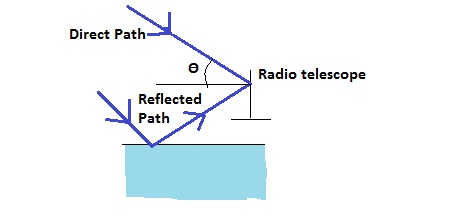Astronomers observe a 68.0-MHz radio source both directly and by reflection from the sea as shown...

Question:

Astronomers observe a 68.0-MHz radio source both directly and by reflection from the sea as shown in the figure.If the receiving dish is 33.0 m above sea level,what is the angle of the radio source above the horizon at the first maximum.Interference:

Interference is the phenomenon in which two waves superimpose each other to produce a wave of greater or lower amplitude. When the wave produced is of greater amplitude than the two waves that superimpose must be in-phase. This process is called constructive interference. On the other hand, the process is called destructive interference when the two waves that superimpose are out-of-phase, thus, producing a wave of lower amplitude.

Given:

{eq}f = 68.0 \ MHz = 68.0 \times 10^6 \ Hz {/eq} Radio source frequency

{eq}c = 3.0 \times 10^8 \frac {m}{s} {/eq} Speed of light

{eq}h = 33.0 \ m {/eq} Height of the receiving dish

First, we can solve for the wavelength of the radio source using the formula given by,

{eq}\lambda = \frac {c}{f} = \frac {3.0 \times 10^{8} \frac {m}{s}}{68.0 \times 10^6 \ Hz} = 4.41176 \ m {/eq}

Taking note that this is a constructive interference from the term maximum, we can solve for the angle of the radio source using the formula in constructive interference given by,

{eq}2 h sin \theta = (m - \frac {1}{2}) \lambda {/eq} where

{eq}m = 1 {/eq}

{eq}2 h sin \theta = (\frac {1}{2}) \lambda {/eq}

Solving for the angle,

{eq}\theta = sin^{-1} \frac {\lambda}{4 h} = sin^{-1} (\frac {4.41176 \ m}{4 (33.0 \ m)}) = \boxed {1.92^\circ} {/eq}